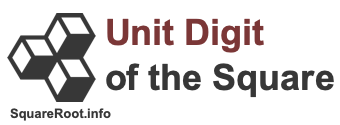Unit digit of the square of 40Here we will show you two ways of finding the unit digit of the square of 40. First, we will define what the unit digit of the square of 40 means. Next, we will find the unit digit of the square of 40 using the Rule Method, and then we will find the unit digit of the square of 40 using the Math Method.

The last digit (one digit) of the product you get when you multiply 40 by itself is the unit digit of the square of 40.

Rule Method
The unit digit of any square will never be 2, 3, 7, or 8. It can be only 0, 1, 4, 5, 6, or 9. Furthermore, the unit digit of the square of a number depends on its units digit. In other words, the unit digit of the square of 40 depends on 0, because that is the last digit in 40. Here are the rules:

Rule A) If the unit digit of a number is 0, then the unit digit of the square of that number is 0.

Rule B) If the unit digit of a number is 1 or 9, then the unit digit of the square of that number is 1.

Rule C) If the unit digit of a number is 2 or 8, then the unit digit of the square of that number is 4.

Rule D) If the unit digit of a number is 5, then the unit digit of the square of that number is 5.

Rule E) If the unit digit of a number is 4 or 6, then the unit digit of the square of that number is 6.

Rule F) If the unit digit of a number is 3 or 7, then the unit digit of the square of that number is 9.

The unit digit of 40 is 0, therefore Rule A applies, and the unit digit of the square of 40 is 0.

Unit digit of the square of 40 = 0

Math Method
The unit digit of the square of a number depends on its units digit with this method too. Here you multiply the unit digit of the number by itself, and then look at the unit digit of that product. Again, the unit digit of 40 is 0, so you multiply 0 by itself, and then look at the unit digit to get the answer once again:

0 × 0 = 0
Unit digit of 0 is 0.
Unit digit of the square of 40 = 0

Of course, instead of using either of the methods above, you can multiply 40 by itself if you have the time or a calculator, and then identify the unit digit to get the answer to the unit digit of the square of 40. We did that below to double-check that our answer is correct:

40 × 40 = 1600
Unit digit of 1600 is 0.
Unit digit of the square of 40 = 0

Unit Digit of the Square Calculator
Please enter another number that we can find the unit digit of the square for.

Unit digit of the square of

Unit digit of the square of 41
Here is the unit digit of the square of the next number on our list.